Mean, median and Mode for Grouped frequency distribution

 Page 3/6 Date 03.05.2017 Size 341.52 Kb. #18702

Mean, median and Mode for Grouped frequency distribution

• Mean,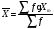where; =class midpoint

(Student must show the working ie. Find midpoint and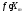)

• Median :

- find cumulative frequency

- find location of median class =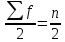- Median :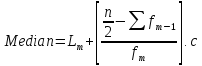Where;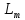=lower boundary of the median class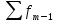= cumulative frequency until the point L (before median class)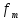= frequency of the median class

c =class width of median class

• Mode :

- find location of modal class : class with the largest frequency

-Mode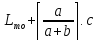where;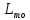=lower boundary of the modal class

a = different between frequencies of modal class and the class before it.

b= different between frequencies of modal class and the class after it.

c =class width of median class

Example:

1. These numbers of books were read by each of the 28 students in a literature class. Find mean, median and mode.
 Number of books Frequency 0 – 2 2 3 – 5 6 6 – 8 12 9 – 11 5 12 – 14 3

1. Eighty randomly selected light bulbs were tested to determine their lifetimes (in hours). This frequency distribution was obtained. Find mean, median and mode.
 Class Boundaries Frequency 52.5 – 63.5 6 63.5 – 74.5 12 74.5 – 85.5 25 85.5 – 96.5 18 96.5 – 107.5 14 107.5 – 118.5 5

3.3.2 MEASURES OF VARIATION

Variance and Standard deviation (the spread of data set)
 Group A Group B 80 55 81 88 82 100 =81 =81 Variation, s2 =1 Variation, s2 =543